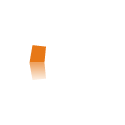pl# Statistical Analysis

Offer:
• computer keying the data collected (for preparing the database for statistical analysis)
• preparation of a database (ordering, detect errors and inaccuracies in the collected material)
• carry out statistical analysis:
• descriptive statistics - variables distributions, frequency, averages, standard deviations, etc.
• basic statistics - correlation matrices, chi-square test, comparing the groups, simple regression,
• advanced statistics, etc. - Regression Models (GLM), models of ANOVA / MANOVA , survival analysis, etc.
• study results:
• interpretation and text description
• preparing tables and graphs
• multimedia presentation data (presentation in MS Power Point presentation on-line flash presentation)